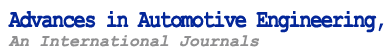Tp_Editing System.E (TES.E)> Volume 1, No. 1 (March 2018) Volume 1, No. 2 (June 2019) Volume 2, No. 1 (2021) CONTENTS Volume 2, Number 1, 2021

Abstract
This paper is related to path planning for a car-like vehicle to park in parallel. The proposed path planning algorithm is based on geometrical equations and kinematic model of the vehicle. The algorithm aims to calculate the path in order to park the vehicle, by first retrieving the vehicle from its final parked position in a MATLAB created environment model. The approach is tested in the environment model with different lateral positions in order to find the working range of the used geometrical equations. Within this scope, two formulas are derived which relate vehicle parameters and measured environmental telemetry to parking path. The first formula states the relation between lateral position and change in orientation angle of the ego vehicle during reversing maneuver. The second formula demonstrates the relation between lateral position and longitudinal position of the ego vehicle when at the start of the reversing maneuvers. By combining the derived equations, a graph is created. For a defined lateral distance, change in orientation angle during reversing maneuvers and longitudinal position at the start of reversing maneuvers of the ego vehicle can be obtained by using this graph.

Key Words
Ackerman steering; automated parking; parallel parking